• 中值滤波原理??中值滤波本质上是一种统计排序滤波器。对于原图像中某点(i，j)，中值滤波以该点为中心的邻域内的所有像素的统计排序中值作为(i，j)点的响应。??中值不同于均值，是指排序队列中位于中间位置的元素的值...
中值滤波原理??中值滤波本质上是一种统计排序滤波器。对于原图像中某点(i，j)，中值滤波以该点为中心的邻域内的所有像素的统计排序中值作为(i，j)点的响应。??中值不同于均值，是指排序队列中位于中间位置的元素的值，例如，采用3x3中值滤波器，某点(i，j)的8个邻域的一系列像素值为12，18，25，11、118，17、35，29，23，统计排序结果为11、12，17，18，23，25，29，35，118。排在中间位置(第5位)的23即作为(i，j)点中值滤波的响应g(i，j)。显然，中值滤波并非线性滤波器。原理图解：选定图像中的某个像素，采用3*3的滤波窗口进行滤波，将周围的8个像素连同选定的像素进行排序，然后选择排好序的中心像素值代替原来的像素值。即将图中的23代替原来的118。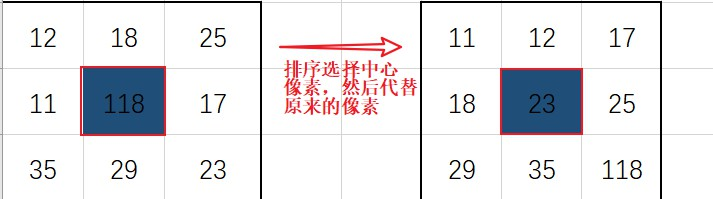图中可以知道，当像素点太大或者太小时都不同意被选中，所以中值滤波对像素值比较大或者比较小的噪声滤除的效果比较好，如：椒盐噪声，对比较均匀的噪声滤除的效果一般，如：高斯噪声。中值滤波的优点：??中值滤波对于某些类型的随机噪声具有非常理想的降噪能力，对于线性平滑滤波而言，在处理的像素邻域之内包含噪声点时，噪声的存在总会或多或少地影响该点的像素值的计算，(对于高斯平滑影响程度同噪声点到中心点的距离成正比)，但在中值滤波中噪声点则常常是直接被忽略掉的；而且同线性平滑滤波器相比，中值滤波在降噪同时引起的模糊效应较低。使用MATLAB展示中值滤波效果：在matlab中中值滤波的函数是medfilt2函数，示例1，中值滤波对椒盐噪声和高斯噪声的滤波效果展示。1、采用的原图是：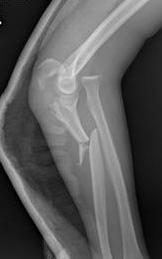2、滤除椒盐噪声和高斯噪声的对比代码如下：%% 滤波效果展示clear; clc; close all;rawimg = imread(‘..picture1.jpg‘);[~,~,index] = size(rawimg);if index ~= 1rawimg = rgb2gray(rawimg);  % 转化为灰度图end% 显示原图figure;imshow(rawimg);title(‘原图‘);% 添加噪声salt_img=imnoise(rawimg,‘salt & pepper‘,0.04);     % 叠加密度为0.04的椒盐噪声guas_img = imnoise(rawimg,‘gaussian‘,0.04);figure;subplot(1,2,1);imshow(salt_img);title(‘添加椒盐噪声‘);subplot(1,2,2);imshow(guas_img);title(‘添加高斯噪声‘);% 进行滤波salt_fit = medfilt2(salt_img,[3 3]);   % 采用二维中值滤波函数对图像滤波，滤波窗口是3*3guas_fit = medfilt2(guas_img,[3,3]);  % 滤除高斯噪声% 滤波效果：figure;subplot(1,2,1);imshow(salt_fit);title(‘椒盐噪声滤波效果‘);subplot(1,2,2);imshow(guas_fit);title(‘高斯噪声滤波效果‘);添加噪声效果示例：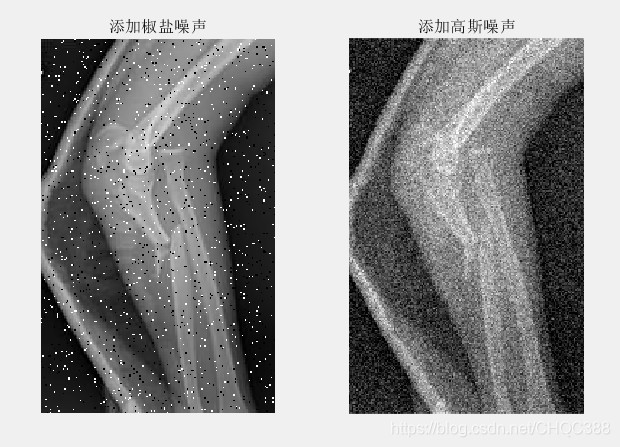使用中值滤波滤除的效果示例：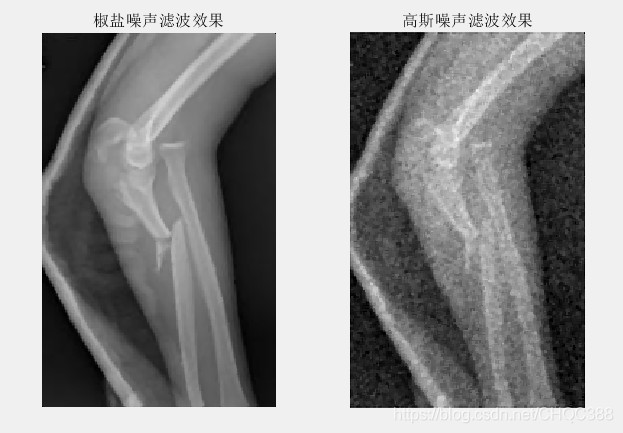分析：可以很明显的看到，中值滤波对椒盐噪声的滤除效果比高斯噪声要好，边缘部分的细节有部分的保留。3、选择不同的窗口对椒盐噪声的滤除效果对比示例代码：%% 滤波窗口选择展示clear; clc; close all;rawimg = imread(‘..picture1.jpg‘);[~,~,index] = size(rawimg);if index ~= 1rawimg = rgb2gray(rawimg);  % 转化为灰度图end% 添加噪声salt_img=imnoise(rawimg,‘salt & pepper‘,0.04);     % 叠加密度为0.04的椒盐噪声figure;subplot(1,2,1);imshow(rawimg);title(‘原图‘);subplot(1,2,2);imshow(salt_img);title(‘添加椒盐噪声‘);% 进行滤波min_fit = medfilt2(salt_img,[3 3]);   % 采用二维中值滤波函数对图像滤波，滤波窗口是3*3max_fit = medfilt2(salt_img,[9,9]);  % 滤除高斯噪声% 滤波效果：figure;subplot(1,2,1);imshow(min_fit);title(‘3*3滤波窗口效果‘);subplot(1,2,2);imshow(max_fit);title(‘9*9滤波窗口效果‘);添加椒盐噪声的效果示例：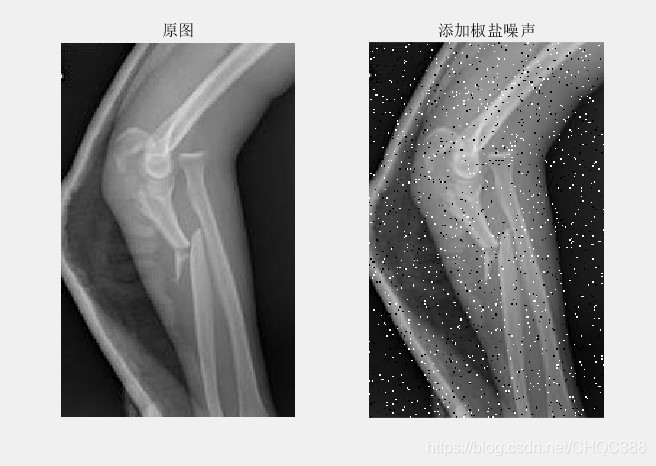分别选择[3,3]滤波窗口和[9,9]滤波窗口对噪声图像进行滤波效果对比示例：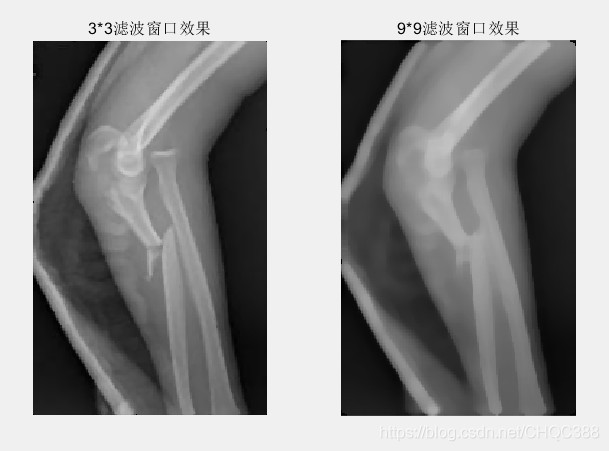分析：可以很明显看到，当中值滤波的窗口选择越大的时候滤波的效果比较明显，但是窗口选择越大则对原图的边缘，细节部分滤除的部分就会越多，这个特性可以根据自己的需要进行调节。
展开全文• 比如下图，左图是没有处理的原图，250在该区域由为突出，通过对3*3的9个数据进行排序，将中间值150重新填入，即滤波完成，原本的噪声点被去掉，该区域恢复平缓。同理，在边缘区域中，对于边界来说，高频不会影响，而...
中值滤波器原理如果不在边缘区域，图像的数据是平缓的，没有太大的差值。因此，一个噪声点的值要么过大，要么过小。比如下图，左图是没有处理的原图，250在该区域由为突出，通过对3*3的9个数据进行排序，将中间值150重新填入，即滤波完成，原本的噪声点被去掉，该区域恢复平缓。同理，在边缘区域中，对于边界来说，高频不会影响，而过低数值将会突出，中值的选择将不会受到影响，除非3*3的整块区域都被污染，这时我们可以考虑更大的核来处理。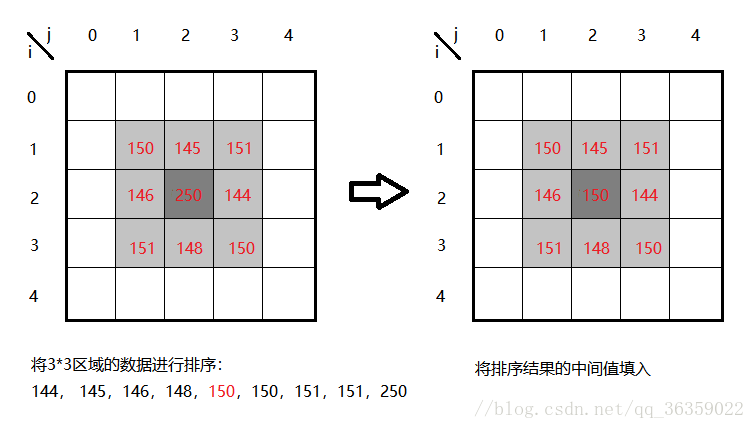java 实现/*** Title: medfilt2* Description:中值滤波 * @param imagesPos* @param tmp 模板矩阵 2*2* @return*/public static  Matrix     medfilt2(Matrix  imagesPos, Matrix  tmp) {/**imagesPos 计算矩阵外围追加数值为0外围，以防矩阵下表越界*/Matrix appendPos=DenseMatrix.Factory.emptyMatrix();appendPos=appendPos.appendVertically(Ret.NEW, imagesPos);Matrix  v= DenseMatrix.Factory.zeros(1,imagesPos.getColumnCount());appendPos=appendPos.appendVertically(Ret.NEW, v);Matrix  h= DenseMatrix.Factory.zeros(imagesPos.getRowCount()+1,1);appendPos=appendPos.appendHorizontally(Ret.NEW, h);Matrix  matRt= DenseMatrix.Factory.zeros(imagesPos.getRowCount(),imagesPos.getColumnCount());for(int i=0;iMatrix  matTmp= DenseMatrix.Factory.zeros(tmp.getColumnCount(), tmp.getColumnCount());for(int j=0;j/**求和*/double [] d= {appendPos.getAsDouble(i,j),appendPos.getAsDouble(i,j+1),appendPos.getAsDouble(i+1,j),appendPos.getAsDouble(i+1,j+1)};Arrays.sort(d);/**依据模板矩阵2*2 计算中值*/double  dbMid=(d+d)/2;matRt.setAsDouble(dbMid, i,j);}}return  matRt;}
展开全文• pixel是通过标准jdk或android bitmap获取的图像32位像素数组这个方法的滤波器尺寸是3*3，需要更大尺寸滤波器可以依此类推，ColorModel可根据各平台变化调整private int[] medianFiltering(int[] pixel, int w, int h...
pixel是通过标准jdk或android bitmap获取的图像32位像素数组这个方法的滤波器尺寸是3*3，需要更大尺寸滤波器可以依此类推，ColorModel可根据各平台变化调整private int[] medianFiltering(int[] pixel, int w, int h) {int[] newPixel = new int[w * h];int[] tempR = new int;int[] tempG = new int;int[] tempB = new int;ColorModel cm = ColorModel.getRGBdefault();// median values of the matrixint r;int g;int b;for (int y = 0; y < h; y++) {for (int x = 0; x < w; x++) {if (x == 0 || x == w - 1 || y == 0 || y == h - 1) {newPixel[y * w + x] = pixel[y * w + x];continue;}tempR = cm.getRed(pixel[x - 1 + (y - 1) * w]);tempR = cm.getRed(pixel[x + (y - 1) * w]);tempR = cm.getRed(pixel[x + 1 + (y - 1) * w]);tempR = cm.getRed(pixel[x - 1 + y * w]);tempR = cm.getRed(pixel[x + y * w]);tempR = cm.getRed(pixel[x + 1 + y * w]);tempR = cm.getRed(pixel[x - 1 + (y + 1) * w]);tempR = cm.getRed(pixel[x + (y + 1) * w]);tempR = cm.getRed(pixel[x + 1 + (y + 1) * w]);r = getMedianValue(tempR);tempG = cm.getGreen(pixel[x - 1 + (y - 1) * w]);tempG = cm.getGreen(pixel[x + (y - 1) * w]);tempG = cm.getGreen(pixel[x + 1 + (y - 1) * w]);tempG = cm.getGreen(pixel[x - 1 + y * w]);tempG = cm.getGreen(pixel[x + y * w]);tempG = cm.getGreen(pixel[x + 1 + y * w]);tempG = cm.getGreen(pixel[x - 1 + (y + 1) * w]);tempG = cm.getGreen(pixel[x + (y + 1) * w]);tempG = cm.getGreen(pixel[x + 1 + (y + 1) * w]);g = getMedianValue(tempG);tempB = cm.getBlue(pixel[x - 1 + (y - 1) * w]);tempB = cm.getBlue(pixel[x + (y - 1) * w]);tempB = cm.getBlue(pixel[x + 1 + (y - 1) * w]);tempB = cm.getBlue(pixel[x - 1 + y * w]);tempB = cm.getBlue(pixel[x + y * w]);tempB = cm.getBlue(pixel[x + 1 + y * w]);tempB = cm.getBlue(pixel[x - 1 + (y + 1) * w]);tempB = cm.getBlue(pixel[x + (y + 1) * w]);tempB = cm.getBlue(pixel[x + 1 + (y + 1) * w]);// median valueb = getMedianValue(tempB);newPixel[y * w + x] = 255 << 24 | r << 16 | g << 8 | b;}}return newPixel;}
展开全文• 来对下面的图像滤波，其实就是对各个像素点进行数学运算的过程均值滤波均值滤波的实现很简单，把滤波器的各个像素点相加在取平均就可以了。public static int getAVEcolor(int x,int y,BufferedImage bi){int color=...
来对下面的图像滤波，其实就是对各个像素点进行数学运算的过程均值滤波均值滤波的实现很简单，把滤波器的各个像素点相加在取平均就可以了。public static int getAVEcolor(int x,int y,BufferedImage bi){int color=0;int r=0,g=0,b=0;for(int i=x-1;i<=x+1;i++)for(int j=y-1;j<=y+1;j++){color=bi.getRGB(i, j);r += (color >> 16) & 0xff;g += (color >> 8) & 0xff;b += color & 0xff;}int ia = 0xff;int ir = (int)(r/9);int ig = (int)(g/9);int ib = (int)(b/9);color = (ia << 24) | (ir << 16) | (ig << 8) | ib;return color;}效果如下图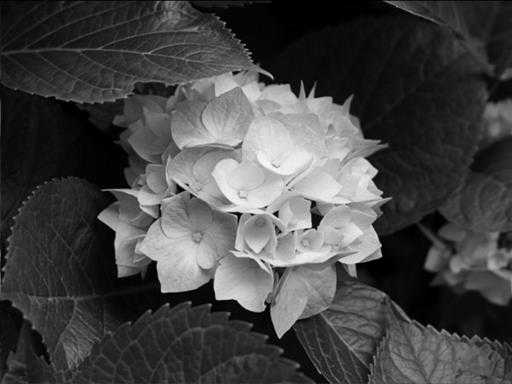中值滤波取滤波器的各个像素点的中值。如3*3的滤波器就取排列后的第5个数public static int getMidcolor(int x,int y,BufferedImage bi){int color=0;int m=0;int a[]=new int;for(int i=x-1;i<=x+1;i++)for(int j=y-1;j<=y+1;j++){color=bi.getRGB(i, j);a[m]=color;m++;}Arrays.sort(a);color=a;return color;}效果如下图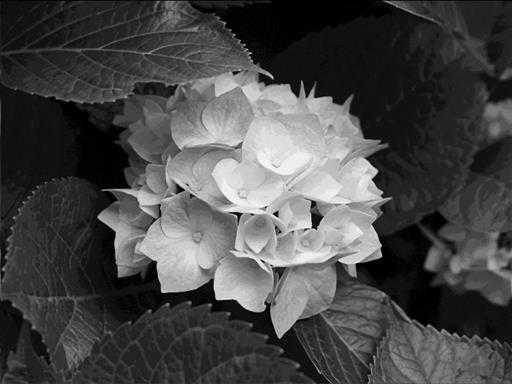拉普拉斯滤波其实也是各个像素点的基本运算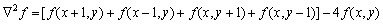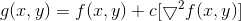//LPLS滤波中间权重为8public static int getLPLScolor8(int x,int y,BufferedImage bi){int color=0,r=0,g=0,b=0;for(int i=x-1;i<=x+1;i++)for(int j=y-1;j<=y+1;j++){if(i!=x&&j!=y){color=bi.getRGB(i, j);r -= (color >> 16) & 0xff;g -= (color >> 8) & 0xff;b -= color & 0xff;}else if(i==x&&j==y){color=bi.getRGB(i, j);r += 8*((color >> 16) & 0xff);g += 8*((color >> 8) & 0xff);b += 8*(color & 0xff);}}color=bi.getRGB(x, y);r += (color >> 16) & 0xff;g += (color >> 8) & 0xff;b += color & 0xff;int ia = 0xff;color = (ia << 24) | (clamp(r) << 16) | (clamp(g) << 8) | clamp(b);return color;}//LPLS中间权重为4public static int getLPLScolor4(int x,int y,BufferedImage bi){int color=0,r=0,g=0,b=0;color=bi.getRGB(x, y+1);r -= (color >> 16) & 0xff;g -= (color >> 8) & 0xff;b -= color & 0xff;color=bi.getRGB(x-1, y);r -= (color >> 16) & 0xff;g -= (color >> 8) & 0xff;b -= color & 0xff;color=bi.getRGB(x+1, y);r -= (color >> 16) & 0xff;g -= (color >> 8) & 0xff;b -= color & 0xff;color=bi.getRGB(x, y-1);r -= (color >> 16) & 0xff;g -= (color >> 8) & 0xff;b -= color & 0xff;color=bi.getRGB(x, y);r += 5*((color >> 16) & 0xff);g += 5*((color >> 8) & 0xff);b += 5*(color & 0xff);int ia = 0xff;color = (ia << 24) | (clamp(r) << 16) | (clamp(g) << 8) | clamp(b);return color;}LPLS权重4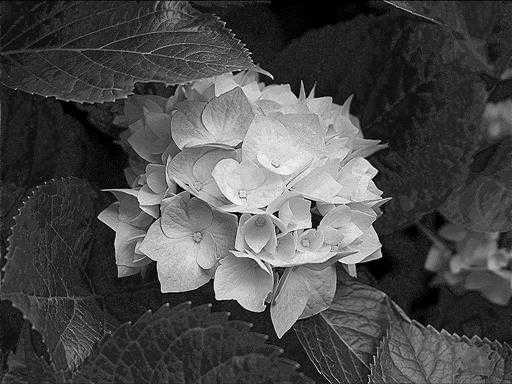LPLS权重8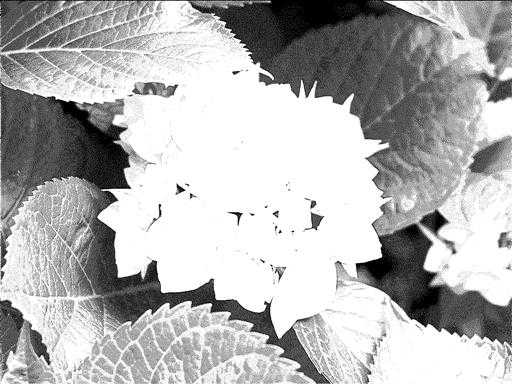Sobel滤波主要用于提取边缘信息，当然也是数学变化public static int getSobelcolor(int x,int y,BufferedImage bi){int color=0;int r1=0,g1=0,b1=0;int r2=0,g2=0,b2=0;int []a1= {-1,-2,-1,0,0,0,1,2,1};int []a2= {1,0,-1,2,0,-2,1,0,-1};int m=0;for(int i=x-1;i<=x+1;i++)for(int j=y-1;j<=y+1;j++){color=bi.getRGB(i, j);r1 += a1[m]*((color >> 16) & 0xff);g1 += a1[m]*((color >> 8) & 0xff);b1 += a1[m]*(color & 0xff);r2 += a2[m]*((color >> 16) & 0xff);g2 += a2[m]*((color >> 8) & 0xff);b2 += a2[m]*(color & 0xff);m+=1;}r1=(int)Math.sqrt(r1*r1+r2*r2);g1=(int)Math.sqrt(g1*g1+g2*g2);b1=(int)Math.sqrt(b1*b1+b2*b2);int ia = 0xff;color = (ia << 24) | (clamp(r1) << 16) | (clamp(g1) << 8) | clamp(b1);return color;}结果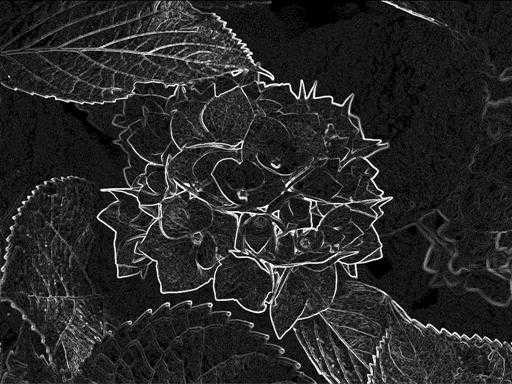他可以用于图像转线稿哎转化后的线稿应该还要去除下噪声才好用注意用java读取的是RGB值，要做位运算转变成在R，G，B的分量。注意转换后的图像的灰度值要小于255大于0，灰度值不在此区间的要让他等于255或0。一开始做LPLS的变化的时候没有注意到这一点，做出来的图像失真很严重，找了半天原因。。。
展开全文• x(mask) 访问在邻域内有效的那些位置，然后您将 median 应用于这些值以检索有效位置上每个邻域的中值 . 但是， nlfilter 已知很慢 . 我建议你看看我的帖子：Matlab Median Filter Code . 这可以非常快速地从第一...
• 目录 来对下面的图像滤波，其实就是对各个像素点进行... 中值滤波 拉普拉斯滤波 Sobel滤波 注意 来对下面的图像滤波，其实就是对各个像素点进行数学运算的过程 均值滤波 均值滤波的实现很简单，把...
• 在讲述如何通过JAVA编程实现中值滤波之前，先谈一下与空间滤波相关的数字图像处理的概念。 1.关于图像和数字图像处理 可以将图像视为是一个二维函数F(x,y),其中(x,y)代表一张数字图像上面对应像素点的坐标，而在...数字图像处理
•图像处理
• 比如下图，左图是没有处理的原图，250在该区域由为突出，通过对3*3的9个数据进行排序，将中间值150重新填入，即滤波完成，原本的噪声点被去掉，该区域恢复平缓。同理，在边缘区域中，对于边界来说，高频不会影响，而...
• ## 中值滤波Java实现

热门讨论 2011-01-24 12:25:23
• 来对下面的图像滤波，其实就是对各个像素点进行数学运算的过程均值滤波均值滤波的实现很简单，把滤波器的各个像素点相加在取平均就可以了。public static int getAVEcolor(int x,int y,BufferedImage bi){int color=...
• ##来对下面的图像滤波，其实就是对各个像素点进行数学运算的过程 [TOC] ###均值滤波均值滤波的实现很简单，把滤波器的各个像素点相加在取平均就可以了。public static int getAVEcolor(int x,int y,BufferedImage bi...
• java实现中值滤波与均值滤波，具体包括通过按钮实现对彩色图像的滤波处理。
• 中值滤波是一种典型的非线性滤波，是基于排序统计理论的一种能够有效抑制噪声的非线性信号处理技术，基本思想是用像素点邻域灰度值的中值来代替该像素点的灰度值，让周围的像素值接近真实的值从而消除孤立的噪声点。...
• http://blog.csdn.net/fastbox/article/details/7984721讨论如何使用卷积作为数学工具来处理图像，实现图像的滤波，其方法包含以下几种，均值滤波，中值滤波，最大最小值滤波，关于什么是卷积以及理解卷积在图像处理...
• 均值滤波和中值滤波的内容非常基础，均值滤波相当于低通滤波，有将图像模糊化的趋势，对椒盐噪声基本无能为力。中值滤波的优点是可以很好的过滤掉椒盐噪声，缺点是易造成图像的不连续性。在下面的代码中，中值滤波...
• 简单介绍一下中值滤波： 数字图像的采样或传输在经过传感器或传输通道时经常受到噪声的干扰。为了便利进一步的图像操作，如：边缘检测、图像分割和模式识别等，有必要甚至是必须对受噪图像进行滤波。中值滤波是由...图像处理
• importjava.awt.*;importjava.awt.event.*;importjava.awt.image.*;importjava.io.File;importjava.io.IOException;importjavax.imageio.ImageIO;importjavax.swing.*;publicclasscolorfilter...
• 方向加权中值滤波算法冯星奎,肖兴明,尹洪君(第二炮兵驻中国工程物理研究院型号办事处,绵阳 621900)摘 要方向加权中值滤波算法是一种新的滤波方法.该方法用于指纹二值化图象处理主要是充分运用指纹方向图和模糊理论...
• 安装OpenCV开发环境，调用直方图均衡化、平滑滤波、中值滤波 关于eclipse的opencv环境配置 运行效果： import org.opencv.core.Core; import org.opencv.core.Mat; import org.opencv.core.Point; import ...opencv 计算机视觉
• 比如下图，左图是没有处理的原图，250在该区域由为突出，通过对3*3的9个数据进行排序，将中间值150重新填入，即滤波完成，原本的噪声点被去掉，该区域恢复平缓。同理，在边缘区域中，对于边界来说，高频不会影响，而...
• 中值滤波方法是，对待处理的当前像素，选择一个模板，该模板为其邻近的若干个像素组成，对模板的像素由小到大进行排序，再用模板的中值来替代原像素的值的方法。 权系数矩阵模板 g...图像处理
• * 功能 对音频数据进行滑动滤波，使其更好的识别 时间：2015/9/11 */ public class MovingAverageFilter { private double[] mBufout = null; private final int mWindowSize = 10; // 移动均值滤波，原始数组...
• [java] view plaincopyprint? "font-family:FangSong_GB2312;font-size:18px;">import java.text.*;  import java.util.*;  import java.io.*;  import javax.servlet.http.*;  import...
• 但是也有一些特别小巧实用易懂的方法，可以用来对数据进行滤波，譬如中值滤波算法。该算法在波形类数据中经常会用上，主要效果是突出特征波形，使得波形更加”凹凸有致“。但是也有一定副作用，那就是如果波形本身就...
• 中值滤波 最大值滤波(也叫作膨胀) 最小值滤波(也叫作腐蚀) 中值滤波 中值滤波的API函数: medianBlur(Mat src, Mat dst, int ksize) src：输入如下 dst：输出图像 ksize：卷积核大小 注意这里的ksize 为单数 ...opencv 计算机视觉
• 但是也有一些特别小巧实用易懂的方法，可以用来对数据进行滤波，譬如中值滤波算法。 　该算法在波形类数据中经常会用上，主要效果是突出特征波形，使得波形更加”凹凸有致“。但是也有一定副作用，那就是如果波形...
• 求一个完成中值滤波的纯JAVA代码。我手里正好有一个C++的中值滤波代码，想对比下两者究竟差距多大。@圆胖肿可否写一个JAVA程序来佐证下你的观点呢？我这面的C++代码，对一张5184*3456的图片，做半径100像素的中值...# java中值滤波java 订阅